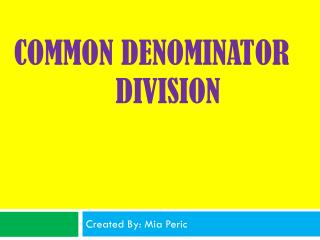DownloadDownload PresentationCommon Denominator Division

# Common Denominator Division

Télécharger la présentation## Common Denominator Division

- - - - - - - - - - - - - - - - - - - - - - - - - - - E N D - - - - - - - - - - - - - - - - - - - - - - - - - - -
##### Presentation Transcript

1. Common Denominator Division Created By: Mia Peric

2. Introduction Dividing fractions is easier than you think. It might seem complicated at first but it will become clear later. In this slideshow I will show you how I learned to divide fractions by whole numbers and fractions by fractions.

3. Fractions by Whole Numbers The way I divide fractions is I switch the numerator and denominator. This is what I do. First I rewrite the question. 6 / 3/5 =

4. Step 2 Next I rewrite the whole number as a fraction. 6 = 6/1

5. Step 3 I switch the numerator and denominator for one fraction. 3/5 = 5/3

6. Step 4 I multiply my new fractions to get the answer. 6/1 * 5/3 = 30/3 = 10

7. Answer The answer to this is 10. 6 / 3/5 = 10